# Splitting and Merging Channels with OpenCV Python

A standard digital Color image is represented by pixels and each pixel is a combination of primary colors. And a channel is a grayscale image that is made up of only one of its primary colors of a colored image. For example, an RGB image has three channels: red, green, and blue.

Observe the below colored images to see how each channel looks separately: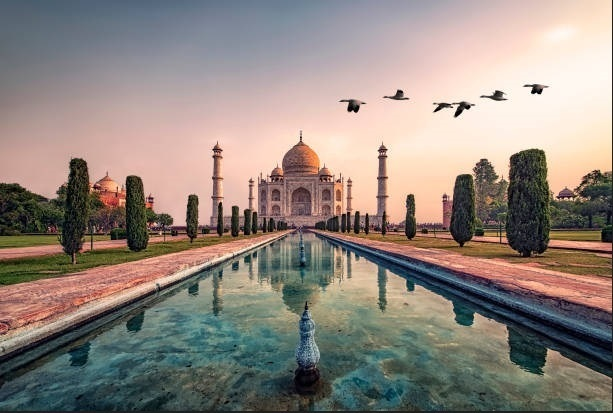Below grayscale images are the representation of each channel of the RGB image.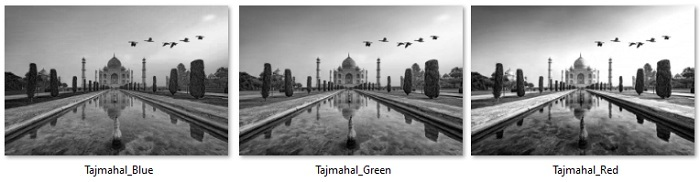In this article, we will discuss how to split and merge channels of an image using python openCV library.

## Splitting channels

Python OpenCV module provides a function cv2.split() to split a multi-channel/colored array into separate single-channel arrays. It will return an array with the three channels, each of which corresponds to blue, green, and red channels represented as a ndarray with two dimensions.

### Syntax

cv2.split(m[, mv])


Where,

• src – input multi-channel array.

• mv – output array or vector of arrays

### Example

In this example, we will take a color image "OpenCV_logo.png" to splitting it into the 3 channels.

import cv2

#split the image into its three channels
(b_channel, g_channel, r_channel) = cv2.split(image)

#display the images
cv2.imshow('blue channel',b_channel)
cv2.imshow('green channel',g_channel)
cv2.imshow('red channel',r_channel)
cv2.waitKey(0)
cv2.destroyAllWindows()


### Input Image### Output ImagesThe color image "OpenCV_logo.png" has been split into three grayscale images: r_channel ("Red channel"), g_channel (Green), b_channel(Blue).

## Merging Channels

The cv2.merge() function takes single-channel arrays and combines them to make a multi-channel array/image. Returns an array of the concatenation of the elements of the input arrays. Following is the syntax of the merge() function –

cv2.merge(mv[, dst])


### Parameters

• mv: input vector of matrices to be merged. all the matrices must have the same size and the same depth.

• count: must be greater than zero. Specifies the number of input matrices when the input vector is a plain C array.

• dst: output array with the same size and the same depth as input array.

### Example

Let us merge the separate blue, green and red channels into a BGR image.

import cv2
#split the image into its three channels
(b_channel, g_channel, r_channel) = cv2.split(image)

#display the images
cv2.imshow('blue channel',b_channel)
cv2.imshow('green channel',g_channel)
cv2.imshow('red channel',r_channel)

# merge the image
image_merged = cv2.merge((b_channel,g_channel,r_channel))
cv2.imshow('merged image',image_merged)
cv2.waitKey(0)
cv2.destroyAllWindows()


### Input Image### Output Image### Example

In this example, we will convert an image to CMYK and then split the channels.

import cv2
import numpy as np

rgbdash = rgb.astype(np.float)/255.

K = 1 -np.max(rgbdash, axis=2)
C = (1-rgbdash [...,2] - K)/(1-K)
M = (1-rgbdash [...,1] - K)/(1-K)
Y = (1-rgbdash [...,0] - K)/(1-K)

# Convert the input BGR image to CMYK colorspace
CMYK = (np.dstack((C,M,Y,K)) * 255).astype(np.uint8)

# Split CMYK channels
Y, M, C, K = cv2.split(CMYK)

# display the images
cv2.imshow("Cyan",C)
cv2.imshow("Magenta", M)
cv2.imshow("Yellow", Y)
cv2.imshow("Key", K)

if cv2.waitKey(0):
cv2.destroyAllWindows()


### Input Image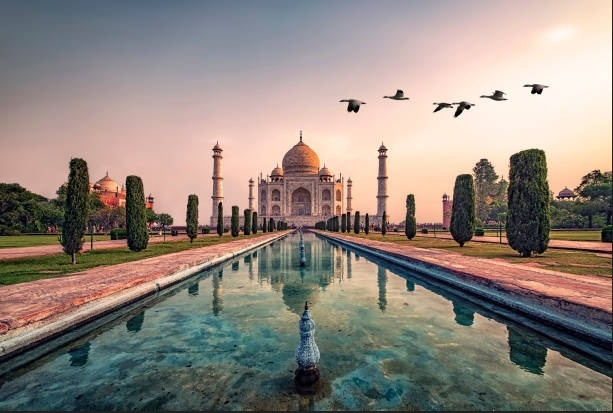### Output Image Cyan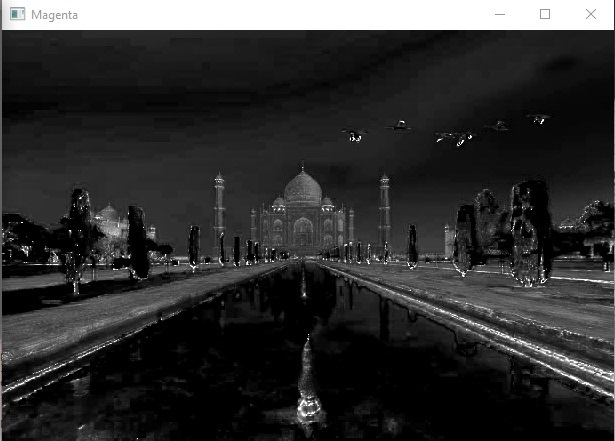### Output Image Magenta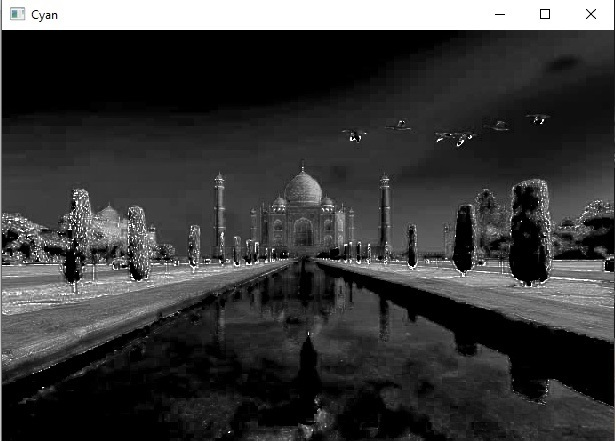### Output Image Yellow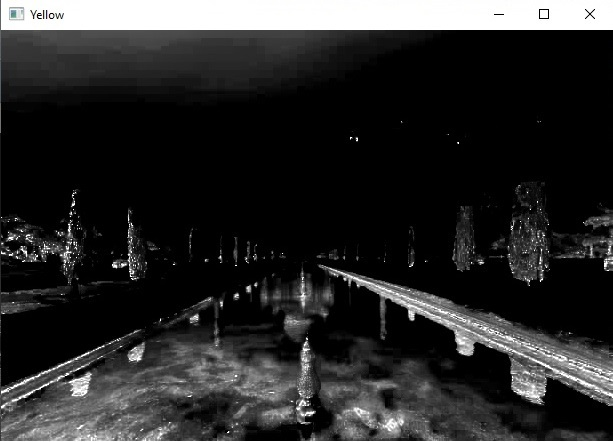### Output Image Key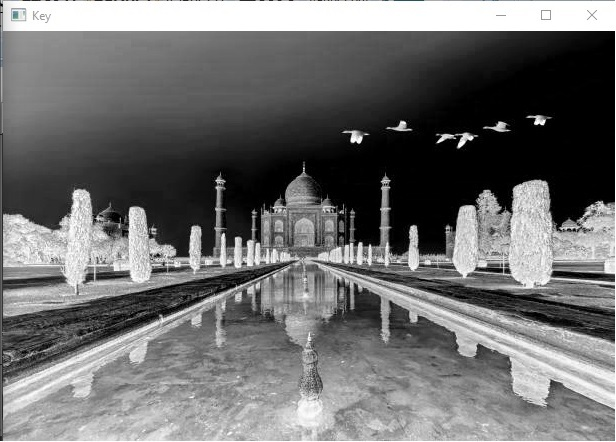In the above example, the RGB image was converted into CMYK and split into four channels Cyan, Magenta, Yellow, and Key.

Updated on: 30-May-2023

619 Views